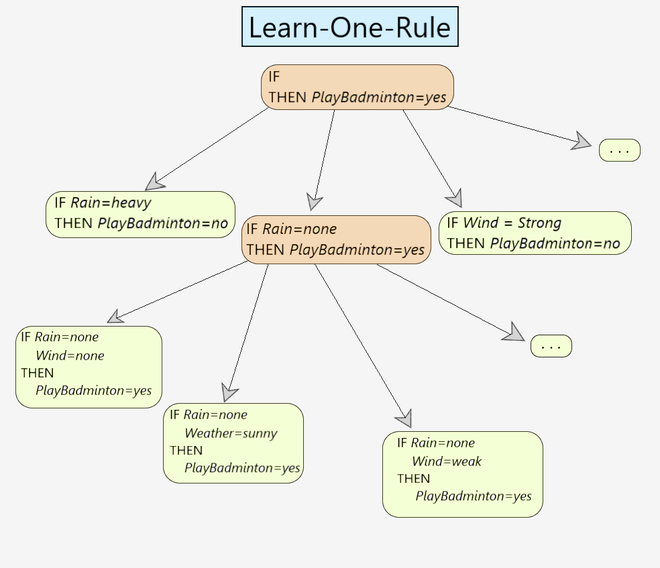Related Articles
Learn-One-Rule Algorithm
• Last Updated : 26 Nov, 2020

Prerequisite: Rule-Based Classifier

Learn-One-Rule:

This method is used in the sequential learning algorithm for learning the rules. It returns a single rule that covers at least some examples (as shown in Fig 1). However, what makes it really powerful is its ability to create relations among the attributes given, hence covering a larger hypothesis space.

```For example:
IF Mother(y, x) and Female(y), THEN Daughter(x, y).
Here, any person can be associated with the variables x and y
```Fig 1: Learn-One-Rule Example

Learn-One-Rule Algorithm

The Learn-One-Rule algorithm follows a greedy searching paradigm where it searches for the rules with high accuracy but its coverage is very low. It classifies all the positive examples for a particular instance. It returns a single rule that covers some examples.

```Learn-One-Rule(target_attribute, attributes, examples, k):

Pos = positive examples
Neg = negative examples
best-hypothesis = the most general hypothesis
candidate-hypothesis = {best-hypothesis}

while candidate-hypothesis:
//Generate the next more specific candidate-hypothesis

constraints_list = all contraints in the form "attribute=value"
new-candidate-hypothesis = all specializations of candidate-
remove all duplicates/inconsistent hypothesis from new-candidate-hypothesis.
//Update best-hypothesis
best_hypothesis = argmax(h∈CHs) Performance(h,examples,target_attribute)

//Update candidate-hypothesis

candidate-hypothesis = the k best from new-candidate-hypothesis
according to Performance.
prediction = most frequent value of target_attribute from examples that match best-hypothesis
IF best_hypothesis:
return prediction

```

It involves a PERFORMANCE method that calculates the performance of each candidate hypothesis. (i.e. how well the hypothesis matches the given set of examples in the training data.

```Performance(NewRule,h):
h-examples = the set of rules that match h
return (h-examples)```

It starts with the most general rule precondition, then greedily adds the variable that most improves performance measured over the training examples.

Learn-One-Rule Example

Let us understand the working of the algorithm using an example:

```Step 1 - best_hypothesis = IF h THEN PlayBadminton(x) = Yes
Step 2 - candidate-hypothesis = {best-hypothesis}
Step 3 - constraints_list = {Weather(x)=Sunny, Temp(x)=Hot, Wind(x)=Weak, ......}
Step 4 - new-candidate-hypothesis = {IF Weather=Sunny THEN PlayBadminton=YES,
Step 5 - best-hypothesis = IF Weather=Sunny THEN PlayBadminton=YES
Step 6 - candidate-hypothesis = {IF Weather=Sunny THEN PlayBadminton=YES,